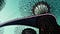# A beginner’s guide to Time series / date functionality

Pandas contains extensive capabilities and features for working with time series data for all domains.

# Timestamps and Periods

Timestamp : references particular moment in time and associates values with data points in time. Timestamped data is the most basic type of time series data that associates values with points in time. For pandas objects it means using the points in time.

# Multiple confusion matrices into one curve: ROC

ROC (Receiver Operator Characteristic) graphs are useful for consolidating the information from a ton of confusion matrices into a single, easy to interpret graph.

The purpose of this article is to serve as an introduction to ROC graphs and we’ll talk about:

• Classifier performance
• ROC space
• Random performance
• Curves in ROC space
• ROC curve in Python

# Classifier performance

A classifier is a mapping from instances to predicted classes. Some classification models produce a continuous output (an estimate of the class membership probability) to which different thresholds may be applied to predict class membership. …

# How learning_curve function from scikit-learn works?

Determines cross-validated training and test scores for different training set sizes.

A cross-validation generator splits the whole dataset k times in training and test data. Subsets of the training set with varying sizes will be used to train the estimator and a score for each training subset size and the test set will be computed. Afterwards, the scores will be averaged over all k runs for each training subset size.

learning_curve(estimator, X, y, groups=None, train_sizes=np.linspace(0.1, 1.0, 5), cv=None, scoring=None, exploit_incremental_learning=False, n_jobs=None, pre_dispatch=”all”, verbose=0, shuffle=False, random_state=None, error_score=np.nan, return_times=False)

Let’s see how learning_curve() do the splits if shuffle=False. To do so, we…

# How do I choose performance metrics for classification algorithm?

Pick a performance metric that reflects the kind of task your algorithm is performing and how you would like your algorithm to behave.Garden by the Bay, Singapore

In classification problems we are working with discrete data and trying to make discrete predictions, therefore, below are some metrics that are widely used in classification problems.

# Confusion matrix

A two-by-two confusion matrix can be constructed representing the dispositions of the set of instances.

# A beginner’s guide to NumPy

This article presents the basics of NumPy , a package for scientific computing with Python.

We’ll cover a few categories of basic array manipulations here:

• Creating NumPy arrays
• Reshaping of arrays
• Math operations with NumPy
• Indexing and slicing of arrays
• Iterating over arrays

First, let’s import NumPy as np. This lets us use the shortcut np to refer to NumPy.